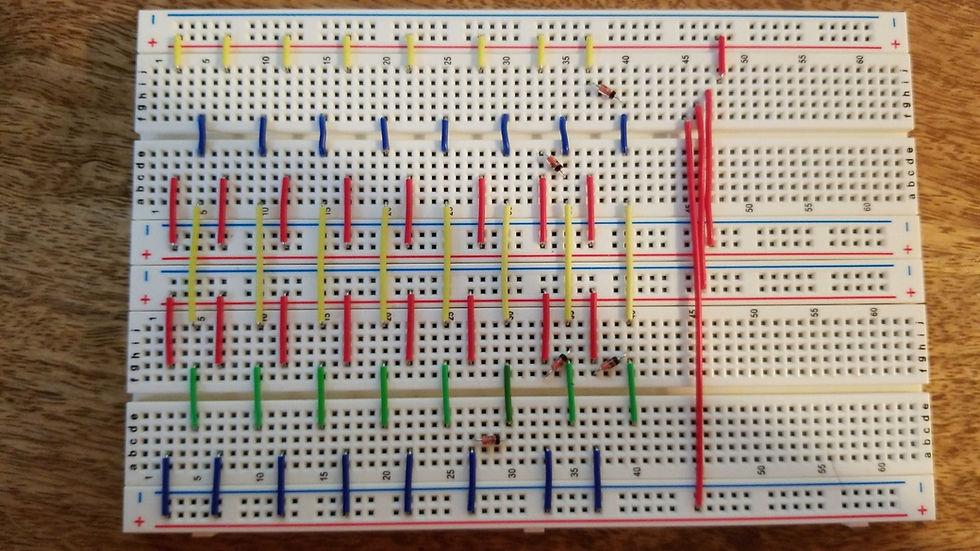top of page
Search
• fezec

# 16 bit Read Only Memory

Updated: Mar 3, 2019

In an effort to wrap my head around the storage and retrieval of binary data, I set out to make a simplistic form of memory- the Read Only Memory or ROM for short. A ROM is the simplest form of memory comprised of (x) addressable units of (n) Registers.

A register is a particular circuit whose sole responsibility is to persist 1 word of data. A word is fixed unit of bit length that can be addressed by a microprocessor. For the purposes of this project 1 word will equal 1 byte of data. Within a register resides individual circuits-each responsible for the retention of its own state. A state can be either on or off (1 or 0). As you may have guessed the individual circuits within a register represent 1 bit. The register simply encapsulates a series of states into a singular unit, aka 1 Word. Additionally, a register exposes the terminals to read/write to the internal bits. The addition of (n) units of registers, within an IC, increases the possible words that can be stored, IE: memory capacity, by a factor of (n).

I find it helpful to liken the internals of memory to that of a Excel spreadsheet. Each row represents a unit of bits that represent 1 word of data. The columns of the table represent a bit and its state (O or 1). Because our table is a Matrix, 1 word of data can be read for each unit simply by referencing (addressing) it.In an excel spreadsheet much like the one in the image seen above, it would be possible to read the first addressable byte by identifying row 1, and reading Columns A thru H. Assuming that each byte represents an integer, and using Row 1 as our first byte of data read, we would find the integer value held was 1. Row 2 represents the number 2, Row 3 represents the number 3, and row 4 retains the number 4.

Remember that each column represents a state either a 0 or 1. The 8 individual values can then be viewed holistically as a unit that make up 1 byte.

While viewing memory as an excel table, it only helps to acknowledge the theory of memory. In the end we're not reading 1's and 0's, nor are we visually processing data. Circuits are concerned with the flow of electrons. 1's and 0's represent whether voltage is present of not. To better witness this, we need to get hands on with the flow of electricity.

The image you see below is the physical representation of our earlier Excel Table from above, making this a useless 4 byte ROM by today's standards. However, its simplicity helps to reveal how memory works.In the image provided above, I have constructed an 8 bit or 1 byte-addressable ROM consisting of the following components:

5 Diodes

Several jumpers

The parts that makeup the ROM are constructed in a very specific fashion as we will soon discuss.

1. The 4 red jumpers provide a means to address a particular byte.

2. The 8 green jumpers represent the output terminals for each bit comprised by the addressable byte.

3. The 5 Diodes are used to signify a stored value of 1

4. The shorter blue, red, and yellow jumpers are used for the individual parallel circuits that represent 8 bits for separate units of registers.

As you may recall from the Excel version of our ROM, each row represented an integer. The first was 1, the second was 2, the third was 3, and the fourth was 4. You may also recall that a cell's value is not the physical representation of 1 or 0. Instead, it's the output of a positive voltage or the lack of one.

By meticulously placing a handful of Diodes, which only allow the flow of electricity in 1 direction within the respective circuits, I have been able to ensure the proper flow of positive voltages to that of the output terminals, in a manner that reflects the aforementioned values of 1, 2, 3, 4.

Using the red jumpers I provide a positive voltage to the 8 parallel circuits that represents an addressable byte. As the power is provided, it will only be able to travel to the output terminals where the Diodes are placed.

Lastly, using another circuit that I had created for another build, we can witness the ROM in action.